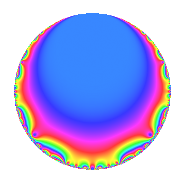# Properties

 Label 83.1.bLevel 83 Weight 1 Character orbit b Rep. character $$\chi_{83}(82,\cdot)$$ Character field $$\Q$$ Dimension 1 Newforms 1 Sturm bound 7 Trace bound 0

# Related objects

## Defining parameters

 Level: $$N$$ = $$83$$ Weight: $$k$$ = $$1$$ Character orbit: $$[\chi]$$ = 83.b (of order $$2$$ and degree $$1$$) Character conductor: $$\operatorname{cond}(\chi)$$ = $$83$$ Character field: $$\Q$$ Newforms: $$1$$ Sturm bound: $$7$$ Trace bound: $$0$$

## Dimensions

The following table gives the dimensions of various subspaces of $$M_{1}(83, [\chi])$$.

Total New Old
Modular forms 2 2 0
Cusp forms 1 1 0
Eisenstein series 1 1 0

The following table gives the dimensions of subspaces with specified projective image type.

$$D_n$$ $$A_4$$ $$S_4$$ $$A_5$$
Dimension 1 0 0 0

## Trace form

 $$q - q^{3} + q^{4} - q^{7} + O(q^{10})$$ $$q - q^{3} + q^{4} - q^{7} - q^{11} - q^{12} + q^{16} - q^{17} + q^{21} + 2q^{23} + q^{25} + q^{27} - q^{28} - q^{29} - q^{31} + q^{33} - q^{37} + 2q^{41} - q^{44} - q^{48} + q^{51} - q^{59} - q^{61} + q^{64} - q^{68} - 2q^{69} - q^{75} + q^{77} - q^{81} + q^{83} + q^{84} + q^{87} + 2q^{92} + q^{93} + O(q^{100})$$

## Decomposition of $$S_{1}^{\mathrm{new}}(83, [\chi])$$ into irreducible Hecke orbits

Label Dim. $$A$$ Field Image CM RM Traces $q$-expansion
$$a_2$$ $$a_3$$ $$a_5$$ $$a_7$$
83.1.b.a $$1$$ $$0.041$$ $$\Q$$ $$D_{3}$$ $$\Q(\sqrt{-83})$$ None $$0$$ $$-1$$ $$0$$ $$-1$$ $$q-q^{3}+q^{4}-q^{7}-q^{11}-q^{12}+q^{16}+\cdots$$